# # 7 指针分析-基础

## # 7.1 指针分析的规则

### # 7.1.1 定义和记号

$p\in V\cup(O\times F)$

$Pointer \subseteq V\cup(O\times F)$

$pt \subseteq Pointer \times P(O)$

### # 7.1.2 规则

$\frac{P_1, P_2, ..., P_m}{Q_1, Q_2, ..., Q_n}$

$\overline{o_i \in pt(x)}$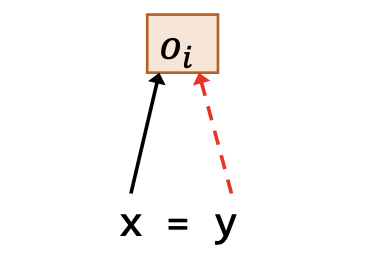$o_j \in pt(o_i.f)$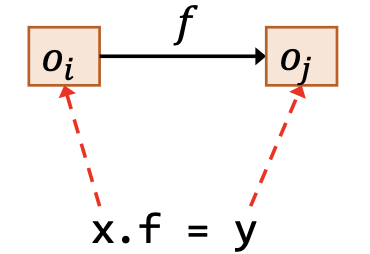$o_j\in pt(y)$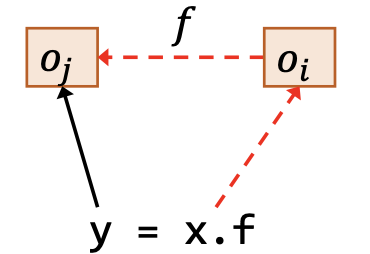## # 7.2 如何实现指针分析

### # 7.2.1 实现思路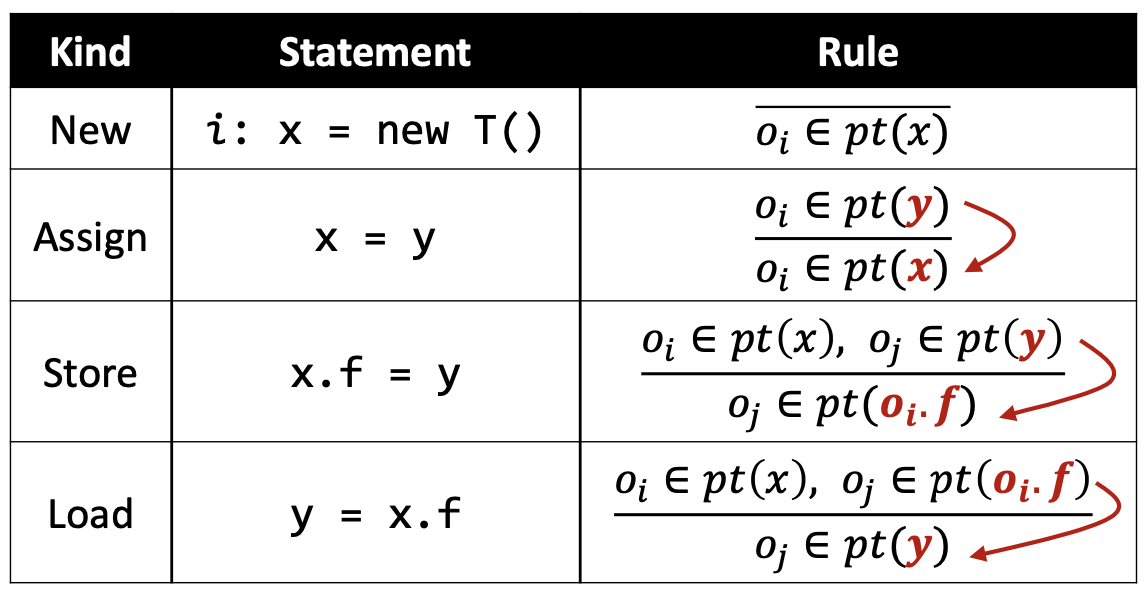• 使用一个图来连接相关的指针；

• $pt(x)$ 改变的时候，将改变的部分传递给 $x$ 的后继们。

### # 7.2.2 指针流图

• PFG中的一个节点 $n$ 代表了一个变量或者某个对象的一个字段，即
$n \in Pointer \subseteq V \cup (O\times F)$
• PFG中的一条边 $x \to y \in Pointer\times Pointer$ 表示指针 $x$ 指向的对象可能会流到指针 $y$ 的指向集合中（即也可能被 $y$ 指向）。

PFG的节点是容易确定的，就是程序中所有的指针；于是，我们只需要根据程序中的语句以及语句对应的规则添加PFG的边即可。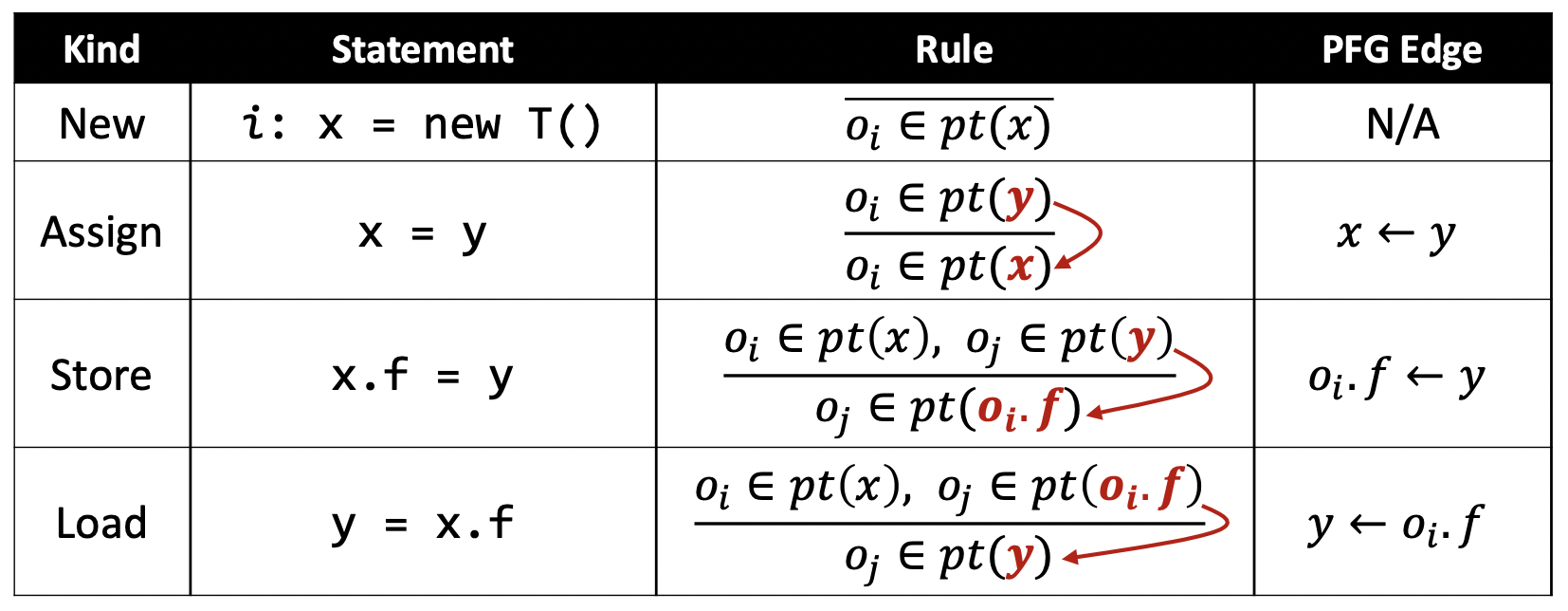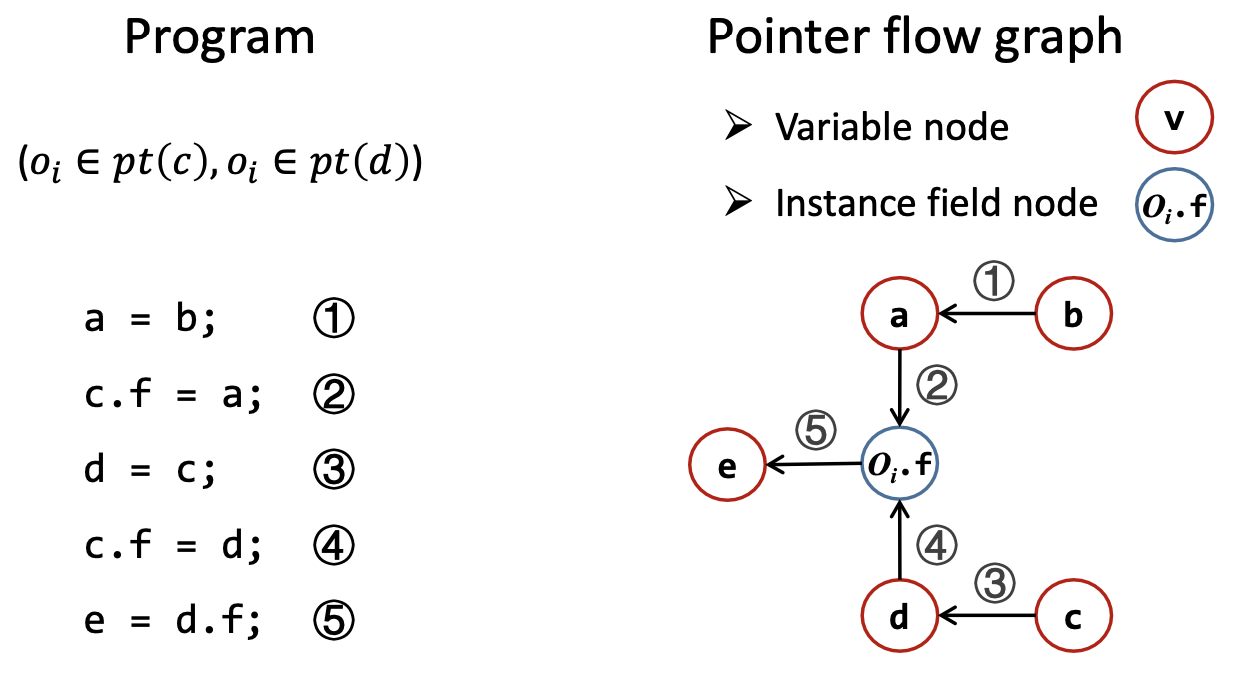### # 7.2.3 分析的步骤

• 构建指针流图 PFG

• 在 PFG 上传递指向信息

## # 7.3 指针分析算法

### # 7.3.2 算法分析

#### # 工作列表

• 工作列表（Worklist）包含了即将被处理的指向信息。
$WL \subseteq Pointer \times P(O)$
• 每个工作列表里面的表项 $(n, pts)$ 是一个指针 $n$ 和 指向集合 $pts$ 的有序对，意味着 $pts$ 应当被传递给 $pt(n)$

$[(x, \{o_i\}), (y, \{o_j, o_k\}), (o_j.f, \{o_l\}), ...]$

#### # 处理 New 和 Assign

1. 如果 $s\to t$ 已经在 PFG 中，则啥也不干，避免冗余操作（第 32 行）

2. 添加 PFG 边（第 33 行）

3. 保证每个 $s$ 指向的对象也被 $t$ 指向（第 34 - 36 行）

1. 如果 $pts$ 是空集，则啥也不干，因为空集就没必要向后传播了（第 41 行）

2. $pts$ 传播给 $n$ 的指向集合 $pt(n)$（第 42 行）

3. $pts$ 传播给 $n$ 在 PFG 上的后继结点（第 43 - 45 行）。

### # 7.3.3 实例

b = new C();
a = b;
c = new C();
c.f = a;
d = c;
c.f = d;
e = d.f;

1
2
3
4
5
6
7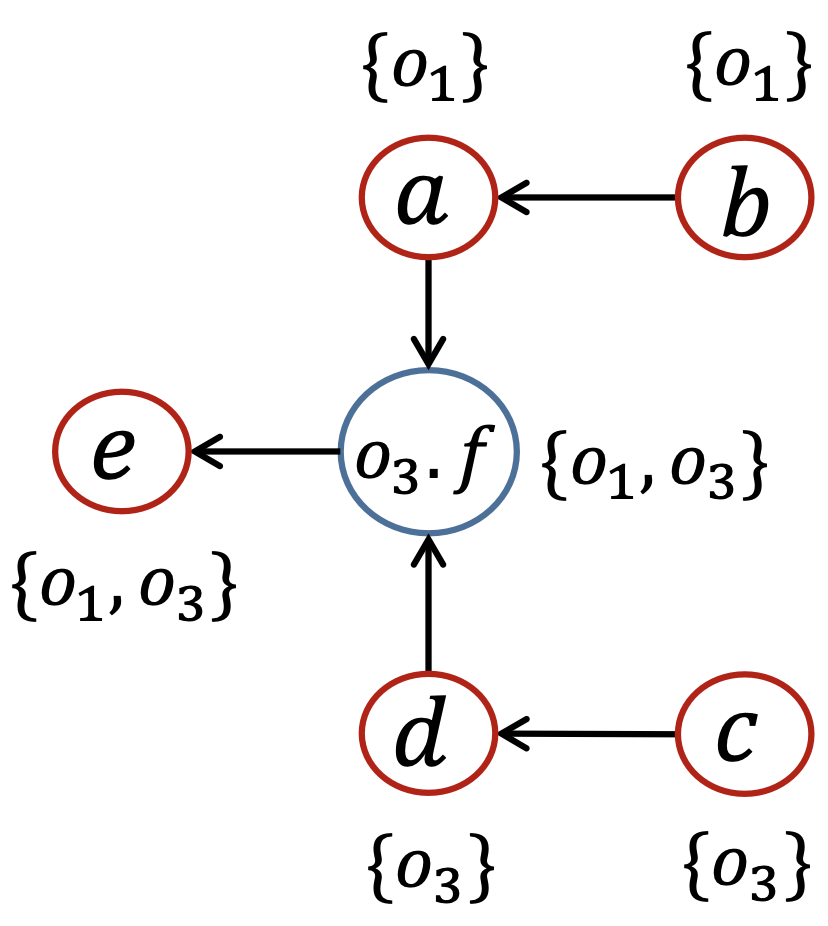## # 7.4 带方法调用的指针分析

### # 7.4.2 调用语句的规则

$o_u\in pt(a_j), 1\le j\le n$
$o_v\in pt(m_{ret})$
$\overline{o_i\in pt(m_{this})}$
$o_u\in pt(m_{p_j}), 1\le j\le n$
$o_v\in pt(r)$
$a_1\to m_{p_1}$
... ...
$a_n\to m_{p_n}$
$m_{ret}\to r$

• $Dispatch(o_i, k)$ 是根据对象 $o_i$ 的类型来做虚方法 $k$ 的派发（详见算法5.1），从而准确的找到对象 $o_i$ 对应的目标方法。这个方法派发是绝对精确的，因为Dispatch算法是对运行时的模拟。这里影响精度的因素只会是变量 $x$ 的指向集合内可能有不止一个指向对象。

• $m_{this}$$m$ 方法对应的 $this$ 变量；

• $m_{p_j}$$m$ 方法的第 $j$ 个形参；

• $m_{ret}$ 是存放 $m$ 方法返回值的变量。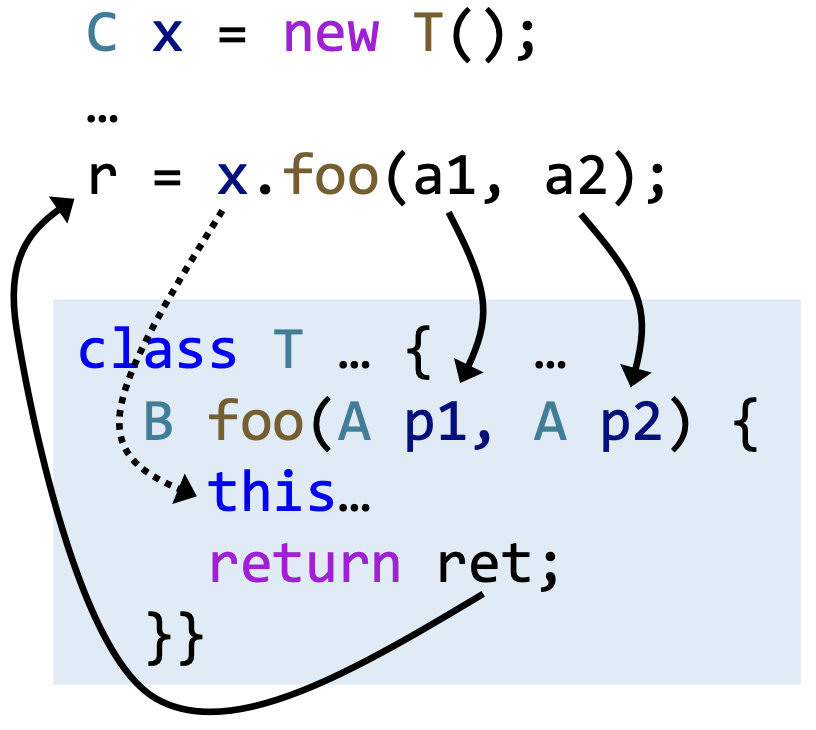### # 7.4.3 过程间的指针分析思路

• 入口方法（比如说 main 方法）是一开始就可达的；

• 其他的可达方法是在分析的过程中不断发现的；

• 只有可达的方法和语句才会被分析。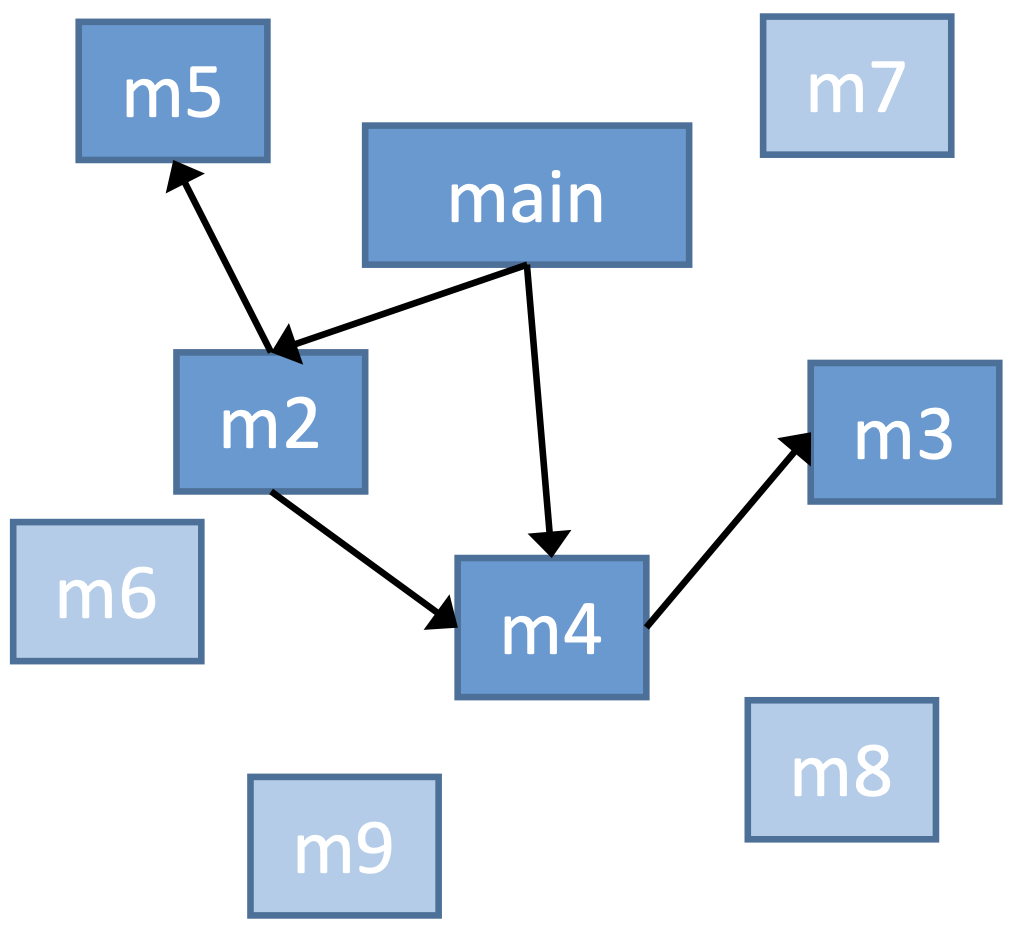### # 7.4.4 算法

#### # 算法分析

1. 添加新的可达的方法和语句（算法第 28 - 30 行）

2. 为新发现的语句更新工作表和指针流图（算法第 31 - 39 行）

• 一开始的时候对入口方法调用（算法第8行）

• 当新的调用边被发现的时候对被发现的新方法调用（算法第 47 - 49 行）

1. 根据接收对象类型以及调用点处的方法签名作方法派发（第 44 - 45 行）

2. 为目标方法的 $this$ 变量添加新的指向关系（第 46 行）

3. 对新发现的目标方法即时（on-the-fly）构建调用图（第 47 - 49 行）

4. 为新发现的目标方法建立传递参数和返回值的 PFG 边（第 50 - 53 行）

#### # 实例

class A {
static void main() {
A a = new A();
A b = new B();
A c = b.foo(a);
}
A foo(A x) {...}
}
class B extends A {
A foo(A y) {
A r = new A();
return r;
}
}

1
2
3
4
5
6
7
8
9
10
11
12
13
14## # 7.5 自检问题

1. 指针分析的规则（Pointer Analysis Rules）是什么？

2. 如何理解指针流图（Pointer Flow Graph）？

3. 指针分析算法（Pointer Analysis Algorithms）的基本过程是什么？

4. 如何理解方法调用（Method Call）中指针分析的规则？

5. 怎样理解过程间的指针分析算法（Inter-procedural Pointer Analysis Algorithm）？

6. 即时调用图构建（On-the-fly Call Graph Construction）的含义是什么？